# Points and Lines

In geometry, points and lines are the fundamental concepts which we need to learn before we learn about different shapes and sizes. A point is a dimensionless shape, since it represents a dot only, whereas a line is a one-dimensional shape. Both points are lines used to draw different shapes and sizes in a plane. These shapes can be two dimensional or three dimensional.

## What is a Point?

A point is a dot in a plane or a piece of paper. A point does not have any length, width or height. It determines the location or position in a plane.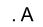Put a dot in a paper and mark it as A or with any capital letter, to show a point. Now if you want to show three different points, then put three dots in a paper and mark them as X, Y and Z. We can read these points as point X, point Y and point Z.

### Types of Points

There are different types of points in geometry, such as:

Collinear points lie on the same line but non-collinear points do not lie on the same line.

Coplanar points line on the same plane but non-coplanar points do not lie on the same plane.

## What is a Line?

A line is a straight path formed by connecting a set of points in a plane. It is a one-dimensional shape that has length but no width and height. A line extends infinitely in both the ends towards opposite directions. We can use upper case letters to denote a line. Also, a line can be marked with a single small letter, such as m, l, etc.

For example, AB is a line but we can also mark it as ‘m’.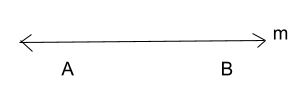### Types of Lines

The different types of lines can be categorized into:

When two lines pass through the same point then they are called intersecting lines. It means the two lines cut or intersect each other at a single point. See the below diagram of intersecting lines.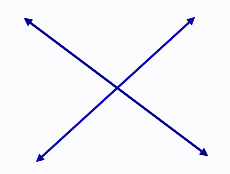When two lines do not meet each other at any point, then they are called parallel lines. The example of parallel lines is a scale or a ruler that has its opposite edges parallel. Another example is the rail lines.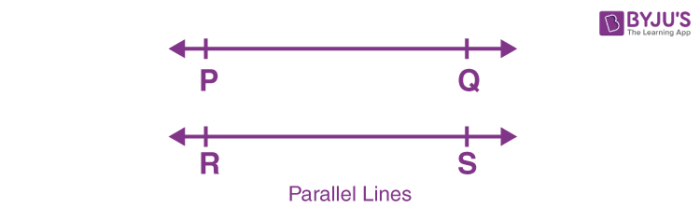Notes: Two lines cannot meet in more than one point Two or more lines can intersect at a single point

## A Line Segment and A Ray

A line segment is a line that has two definite end points. If a line segment has two endpoints A and B, then it is denoted by AB bar.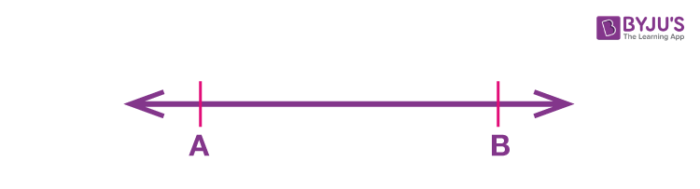A ray is a line that starts from a point and extends indefinitely in a direction. It has only one endpoint.

The example of a ray is a torch light that starts from an end and goes endlessly in one direction.

### Practice Problems

1. Mark the points in a plane: Point A, Point B and Point C.
2. Mark the points A, B and C, that are collinear.
3. Give examples of points that are non-collinear.
4. Draw intersecting lines AB and CD, and mark the intersection point as O.
5. Two lines l and m are parallel to each other. Draw the two lines.

## Frequently Asked Questions on Points and Lines

### What is the point?

A point is a dot marked on a plane that represents the position.

### What is a line?

A line is a series of points that are connected by a straight path and extend infinitely in the opposite directions.

### What are points on the same line called?

The points on the same line are called collinear points.

### How many points make a line?

We need at least two points to determine the line.

### Do lines have endpoints?

Lines do not have endpoints. They extend endlessly in the opposite direction.

### What are the properties of points?

A point has zero size. It is dimensionless. It does not have length, width and height.